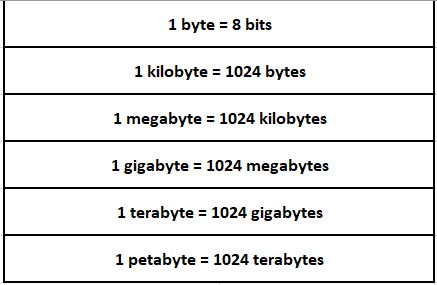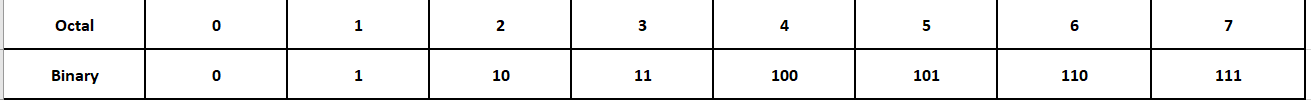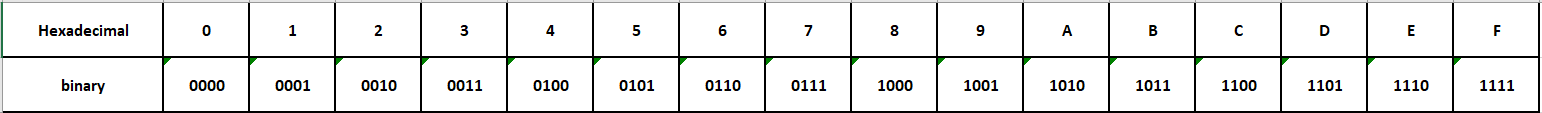In this tutorial, we are going to learn about the various number systems.

## Introduction:

In computers, we normally use four different numbering systems – Decimal, Binary, Octal, and Hexadecimal.

The decimal system is a number system that is used in our day-to-day applications like business, etc. In this system the symbols 0,1,2,3,4,5,6,7,8,9 are used to denote various numbers.

In the binary number system, 0’s and 1’s are the only symbols that are used to represent numbers of all magnitudes. For example, a normal decimal number 7 (seven) is represented in binary as 111. The binary system is mostly used in computers and other computing devices.

A number in a particular base is written as (Number)base. For example, (17)10 is a decimal number (Seventeen), and (10001)2 is a binary number 10001 which actually represents a decimal number whose value is 17.

Since the decimal number system is more commonly used the decimal number (35)10 is simply written as 35. However, if the same number has to be represented in the binary system, it is written as (100011)2.

Similarly, the octal number system uses 8 as its base. It is generally used to display digits and in representing file permissions under UNIX/Linux Operating Systems.

Hexadecimal system or Hex is a number system that uses 16 as a base to represent numbers.

## 1. Decimal Numbers:

The numbers that we use daily belong to the Decimal System. For example, 0,1,2,3,4,…..,9999,…..etc. It is also called a base – 10 system.

It is called the base – 10 number system because it uses 10 unique digits from 0 to 9 to represent any number.

A base (also called the radix) is the number of unique digits or symbols (including 0) that are used to represent a given number.

In a decimal system (where the base is 10), a total of 10 digits (0,1,2,3,4,5,6,7,8, and 9) are used to represent a number of any magnitude. For example, One hundred and Thirty-Five is represented as 135, where

135 = (1 * 10 2) + (3 * 10 1) + (5 * 10 0)

135 = (1 * 100) + (3 * 10) + (5 * 1)

In a similar way, fractions are represented with base – 10 being raised to a negative power.

## 2. Binary Numbers:

The binary number system is used both in mathematics and digital electronics. The binary number system or base – 2 numeral system represents numeric values using only two symbols – Zero (0) and One (1).

Computers have circuits (logic gates) that can be either of the two states: OFF or ON. These two states are represented by Zero (0) and One (1) respectively. It is for this reason that computation in systems is performed using a binary number system (base – 2) where all numbers are represented using 0’s and 1’s.

Each binary digit, i.e., Zero (0) or One (1) is called a bit (known as a binary digit). A collection of 8 such bits is called a Byte.

In computer terminology, different names have been given to multiples of 210 ( i.e., 1024 times the currently existing value ), as shown in the table given below:In computer text, images, music, videos, or any type of data is eventually stored in binary format on the disk.

In the binary system, a total of 2 digits (0 and 1) are used to represent a number of any magnitude.

For example, Zero is represented as 0, where

0 = (0 * 2 0) = (0 * 1)

Similarly, One is represented as 1, where

1 = (1 * 2 0) = (1 * 1)

Now, let us represent the following numbers in binary format:

Two (2): Since 0 and 1 are the only digits the can be used to represent 2, let’s divide 2 by 2 write the quotient and remainder as follows:

[quotient][remainder], i.e., : 

2 = (1 * 2 1) + (0 * 2 0 ) = (2) + (0)

Three (3): Since 0 and 1 are the only digits the can be used to represent 3, let’s divide 3 by 2 write the quotient and remainder as follows:

[quotient][remainder], i.e., : 

3 = (1 * 2 1) + (1 * 2 0 ) = (2) + (1)

Seventeen (17): Since 0 and 1 are the only digits the can be used to represent 17, let’s divide 17 by 2 write the quotient and remainder as follows:

[quotient][remainder], i.e., :, by repeating the above logic for 8 (8 = ), 4(4 = ),2(2 = ) ,and 1(1 = ),  we finally get .

17 = (1 * 2 4) + (0 * 2 3) + (0 * 2 2) + (0 * 2 1) + (1 * 2 0)

17 = (16)        +  (0)         + (0)          +  (0)         +  (1)

In C, binary numbers are prefixed with a leading 0b (or 0B) (digit zero followed by char ‘b’). For example, to store a binary value of four into a variable binary_Four, we write

``int binary_Four = 0b100;``

## 3. Octal Numbers:

The numbering system which uses base – 8 is called the Octal system. A base (also known as radix) is the number of unique digits or symbols (including 0) that are used to represent a given number.

In the octal system (or the base – 8 system), a total of 8 digits (0, 1, 2, 3, 4, 5, 6, and 7) are used to represent a number of any size (magnitude).

For example, Zero is represented as 0, where

0 = (0 * 8 0) = (0 * 1)

Similarly, numbers 1, 2,…, and 7 are represented below:

1 = (1 * 8 0) = (1 * 1)

2 = (2 * 8 0) = (2 * 1)

……

7 = (7 * 8 0) = (7 * 1)

Now, let us represent the following numbers in the octal system:

Eighteen (18): Since 0 to 7 are only digits that can be used to represent 18, let us divide 18 by 8 and write the quotient and remainder as follows:

[quotient][remainder], i.e.,: 

18 = (2 * 8 1) + (2 * 8 0) = (16) + (2)

Four Hundred and Twenty-One (421): Since 0 to 7 are only digits that can be used to represent 421, let us divide 421 by 8 and write the quotient and remainder as follows:

[quotient][remainder], i.e.,:   (further dividing 52 by 8 we get ), which is 

421 = (6 * 8 2) + (4 * 8 1) + (5 * 8 0) = (384) + (32) + (5)

In order to differentiate from decimal numbers, octal numbers are prefixed a leading 0 (zero).

For example, to store an octal value of seven into a variable octal_Seven, we write

``````int octal_Seven = 07;
``````

Similarly, if we want to store an octal representation of a decimal number 9 in a variable number_Nine, we write

``````int number_Nine = 011;
``````

The largest digit in the octal system is (7)8. Number (7)8 in binary is represented as (111)2. In the binary system, three binary digits (bits) are being used to represent the highest octal digit. While converting an octal number to a binary number, three bits are used to represent each octal digit.

The following table shows the conversion of each octal digit into its corresponding binary digits.For example, an octal number 0246 is converted to its corresponding binary form as

``````Octal number      ->   2       4       6
Binary number   ->     010     100    110``````

Hence 0246 is (010100110)2.

Similarly, while converting a binary number into its octal form, the binary number is divided into groups of 3 digits each, starting from the extreme right side of the given number. Each of the three binary digits is replaced with their corresponding octal digits.

If the group of binary digits to the extreme left side of the number do not have three digits, the required number of zeros are added as a prefix to get three binary digits. For example, let us convert a binary number 1101100 into its corresponding octal number.

``````Binary Number      ->    1     101     100
Binary Number      ->    001   101     100  //After prefixing zeros on the extreme left side of the group
Octal Number        ->   1      5       4``````

Hence, the octal equivalent of the given binary 1101100 is 0154.

The number system which uses base – 16 is called hexadecimal system or simply hex. A base (also known as radix) is the number of unique digits or symbols (including 0) that are used to represent a given number.

In the hexadecimal system (or base – 16 number system), a total of 16 symbols are used. Digits from 0 (zero) to 9 (nine) are used to represent values from 0 to 9 respectively and alphabets A, B, C, D, E and F (or a, b, c, d, e, and f) are used to represent values from 10 to 15 respectively.

In many programming languages 0x is used as a prefix to denote a hexadecimal representation.

For example, in the hexadecimal number system, the value of zero is represented as 0x0, where

0 = (0 * 16 0) = (0 * 1)

Similarly,

1 = (1 * 16 0) = (1 * 1)

2 = (2 * 16 0) = (2 * 1)

…..

15 = F = (15 * 16 0) = (15 * 1)

Now, let us represent the following numbers in the hexadecimal system:

Decimal number Eighteen (18):

Since one can use only 0 to 9 and alphabets A to F to represent 18.

Let’s divide 18 by 16 and write the quotient and remainder as follows:

[quotient][remainder], i.e., 

18 = 0x12 = (1 * 161) + (2 * 16 0) = (16) + (2)

One Hundred and Sixty (160).

Since one can use only 0 to 9 and alphabets A to F to represent 160.

Let’s divide 160 by 16 and write the quotient and remainder as follows:

[quotient][remainder], i.e., , [A] (since 10 is represented by A)

160 = 0xA0 = (10 * 161) + (0 * 16 0) = (160) + (0)

Note that both uppercase and lowercase letters can be used when representing hexadecimal values. For example,

``int hex_Hundred_and_Sixty = 0xA0; // or 0Xa0,however 0xA0 is preferred.``

The highest digit in hex is (F)16. The number (F)16 in binary is represented as (1111)2. Here, four binary digits (bits) are used to represent the highest hexadecimal digit. In hex to binary conversion, four bits are used to represent each hex digit.

The following table shows the conversion of each hex digit into its corresponding binary digits.For example, hexadecimal number 0x5AF6 is converted into its corresponding binary form as follows:

``````Hex Number         ->   5     A     F     6
Binary Number      ->  0101  1010  1111  0110``````

Hence, 0x5AF6 is (0101101011110110)2.

Similarly while converting a binary into hex number, the binary number is the first divided into groups of 4 digits each starting from the extreme right side. Each of the four binary digits is replaced with their corresponding hex digits.

If the group to the extreme left side of binary digits does not have four digits, the required number of zeros are added as a prefix to make a group of four binary digits.

For example, let us convert the following binary 1101100 number into hex.

``````Binary Number     ->  110    1100
Binary Number     ->  0110   1100 //After prefixing zeros in the left-most group
Hexal number       ->   6     C``````

Hence, the hex equivalent of the given binary 1101100 is 0x6C.

## References:

Happy Learning 🙂

### About the Author: Hemanth Krishna Vineel RamayanamI’m an Undergraduate Student at the Indian Institute of Information Technology, Design, and Manufacturing, Kurnool, Andhra Pradesh. I belong to the CSE domain.